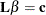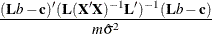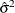The SYSLIN Procedure

STEST Statement

• STEST equation , …, equation / options;

The STEST statement performs an F test for the joint hypotheses specified in the statement.

The hypothesis is represented in matrix notation asand the F test is computed aswhere b is the estimate of, m is the number of restrictions, andis the system weighted mean squared error. See the section Computational Details for information about the matrix.

Each hypothesis to be tested is written as a linear equation. Parameters are referred to as label.variable, where label is the model label and variable is the name of the regressor to which the parameter is attached. (If the MODEL statement does not have a label, you can use the dependent variable name as the label for the model, provided the dependent variable uniquely labels the model.) Each variable name used must be a regressor in the indicated MODEL statement. The keyword INTERCEPT is used to refer to intercept parameters.

STEST statements can be given labels. The label is used in the printed output to distinguish different tests. Any number of STEST statements can be specified. Labels are specified as follows:

• label : STEST …;

The following is an example of the STEST statement:

   proc syslin data=a 3sls;
endogenous y1 y2;
instruments x1 x2;
model y1 = y2 x1 x2;
model y2 = y1 x2;
stest y1.x2 = y2.x2;
run;


The test performed is exact only for ordinary least squares, given the OLS assumptions of the linear model. For other estimation methods, the F test is based on large sample theory and is only approximate in finite samples.

If RESTRICT or SRESTRICT statements are used, the tests computed by the STEST statement are conditional on the restrictions specified. The validity of the tests can be compromised if incorrect restrictions are imposed on the estimates.

The following are examples of STEST statements:

   stest a.x1 + b.x2 = l;
stest 2 * b.x2 = c.x3 + c.x4 ,
a.intercept + b.x2 = 0;
stest a.x1 = c.x2 = b.x3 = 1;
stest 2 * a.x1 - b.x2 = 0;


The PRINT option can be specified in the STEST statement after a slash (/):

PRINT

prints intermediate calculations for the hypothesis tests.

Note: The STEST statement is not supported for the FIML estimation method.